# Maharashtra Board Class 5 Maths Solutions Chapter 5 Fractions Problem Set 18

Balbharti Maharashtra Board Class 5 Maths Solutions Chapter 5 Fractions Problem Set 18 Textbook Exercise Important Questions and Answers.

## Maharashtra State Board Class 5 Maths Solutions Chapter 5 Fractions Problem Set 18

Convert the given fractions into like fractions.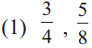Solution :
8 is the multiple of 4 So, make 8, the common denominator $$\frac{3}{4}=\frac{3 \times 2}{4 \times 2}=\frac{6}{8}$$.Thus 6/8 and 5/8are the required like fractions.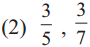Solution :
The number 35 is a multiple of both 5 and 7 So, making 35 as the common denominater $$\frac{3}{5}=\frac{3 \times 7}{5 \times 7}=\frac{21}{35}, \frac{3}{7}=\frac{3 \times 5}{7 \times 5}=\frac{15}{35}$$ Therefore, 21/35 and 15/35 are required like fractions.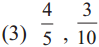Solution :
Here 10 is the multiples of 5. So make 10 as the common denominator $$\frac{4}{5}=\frac{4 \times 2}{5 \times 2}=\frac{8}{10}$$. Thus 8/10 and 3/10 are required like fractions.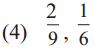Solution :
Least common multiple of 9 and 6 is 18. So, make, 18 as the common denominator. $$\frac{2}{9}=\frac{2 \times 2}{9 \times 2}=\frac{4}{18}, \frac{1}{6}=\frac{1 \times 3}{6 \times 3}=\frac{3}{18}$$. Thus, 4/18 and 3/18 are the required like fractions.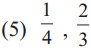Solution :
Least common multiple of 4 and 3 is 12 So, make 12 as common denominator $$\frac{1}{4}=\frac{1 \times 3}{4 \times 3}=\frac{3}{12}, \frac{2}{3}=\frac{2 \times 4}{3 \times 4}=\frac{8}{12}$$. so, $$\frac{3}{12}, \frac{8}{12}$$ are required like fractions.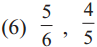Solution :
Least common multiple of 6 and 5 is 30 So, make 30 as common denominator $$\frac{5}{6}=\frac{5 \times 5}{6 \times 5}=\frac{25}{30}, \frac{4}{5}=\frac{4 \times 6}{5 \times 6}=\frac{24}{30}$$ So, $$\frac{25}{30}, \frac{24}{30}$$ are required like fractions.Solution :
Least common multiple of 8 and 6 is 24 So, make 24 as common denominator $$\frac{3}{8}=\frac{3 \times 3}{8 \times 3}=\frac{9}{24}, \frac{1}{6}=\frac{1 \times 4}{6 \times 4}=\frac{4}{24}$$ So, $$\frac{9}{24}, \frac{4}{24}$$ are required like fractions.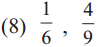Solution :
Least common multiple of 6 and 9 is 18 So, make 18 as common denominator $$\frac{1}{6}=\frac{1 \times 3}{6 \times 3}=\frac{3}{18}, \frac{4}{9}=\frac{4 \times 2}{9 \times 2}=\frac{8}{18}$$ So, 3/18 and 8/18 are the required like fractions.Comparing like fractions
Example (1) A strip was divided into 5 equal parts. It means that each part is 1/5 . The coloured part is $$\frac{3}{5}=\frac{1}{5}+\frac{1}{5}+\frac{1}{5}$$.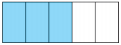The white part is $$\frac{2}{5}=\frac{1}{5}+\frac{1}{5}$$. The coloured part is bigger than the white part. This tells us that 3/5 is greater than 2/5. This is written as 3/5 > 2/5.

Example (2) This strip is divided into 8 equal parts. 3 of the parts have one colour and 4 have another colour. Here, 3/8 < 8/4.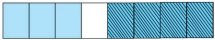In like fractions, the fraction with the greater numerator is the greater fraction.

Comparing fractions with equal numerators
You have learnt that the value of fractions with numerator 1 decreases as the denominator increases.

Even if the numerator is not 1, the same rule applies so long as all the fractions have a common numerator. For example, look at the figures below. All the strips in the figure are alike.
2 of the 3 equal parts of the strip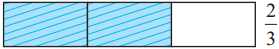2 of the 4 equal parts of the strip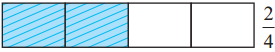2 of the 5 equal parts of the stripThe figure shows that 2/3 > 2/4 > 5/2.Of two fractions with equal numerators, the fraction with the greater denominator is the smaller fraction.

Comparing unlike fractions
Teacher : Suppose we have to compare the unlike fractions 3/5 and 4/7. Let us take an example to see how this is done. These two boys are standing on two blocks. How do we decide who is taller?

Sonu : But the height of the blocks is not the same. If both blocks are of the same height, it is easy to tell who is taller.

Nandu : Now that they are on blocks of equal height, we see that the boy on the right is taller.

Teacher : The height of the boys can be compared when they stand at the same height. Similarly, if fractions have the same denominators, their numerators decide which fraction is bigger.

Nandu : Got it! Let’s obtain the same denominators for both fractions.

Sonu : 5 × 7 can be divided by both 5 and 7. So, 35 can be the common denominator.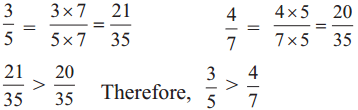To compare unlike fractions, we convert them into their equivalent fractions so that their denominators are the same.Fractions Problem Set 18 Additional Important Questions and Answers

Question 1.
$$\frac{5}{9}, \frac{17}{36}$$
Solution :
36 is the multiple of 9 So, make 36 the common denominator $$\frac{5}{9}=\frac{5 \times 4}{9 \times 4}=\frac{20}{36}$$, Thus 20/36 and 17/36 are the required like fractions.

Question 2.
$$\frac{5}{6}, \frac{7}{9}$$
Solution:
Least common multiple of 6 and 9 is 18 So, make 18 as the common denominator $$\frac{5}{6}=\frac{5 \times 3}{6 \times 3}=\frac{15}{18}, \quad \frac{7}{9}=\frac{7 \times 2}{9 \times 2}=\frac{14}{18}$$ So, 15/18 and 14/18 are the required like fractions.Question 3.
$$\frac{7}{11}, \frac{3}{5}$$
Solution:
Least common multiple of 11 and 5 is 55 So, make 55 as the common denominator. $$\frac{7}{11}=\frac{7 \times 5}{11 \times 5}=\frac{35}{55}, \frac{3}{5}=\frac{3 \times 11}{5 \times 11}=\frac{33}{55}$$. Thus 35/55 and 33/55 are required like fractions.

Scroll to Top